Have any Questions? +01 123 444 555

Motivation for Flow Measurement

# Motivation for Flow Measurement

The combustion process is strongly influenced by intake flow quantity and quality. Therefore these factors are of considerable importance in engine development and optimization aiming on increased efficiency and minimized pollutant emission. Flow Measurements on Momentum FTBs offer a way to determine characteristics, summarizing the fluidic properties of intake and outlet ports as well as peripheral air guiding components. Measurements on PIV FTBs provide detailed insight into the flow entering the cylinder by means of visualized velocity fields (suitable for comparison with CFD). Also flow characteristics can be derived from the velocity fields to simplify comparison between samples.

### The general purposes of flow measurement on combustion engines and peripherals can be outlined as follows:

• Optimization of gas exchange in internal combustion engines
• Combustion control
• Increasing efficiency
• Reducing emissions
• Parameter identification and optimization of peripheral components
• Validation of simulation results
• Quality check
• Development work in terms of
• Quantity (Efficiency)
• Quality (Swirl/Tumble)
• Homogenous distribution

### Units Under Testing:

• Intake and exhaust ports
• Sucking system
• Exhaust system
• Intercooler
• Pipes
• Air filter
• Air mass flowmeter
• Exhaust system Testing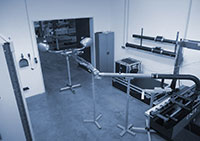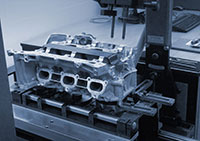• Catalyst and Particular Filter Testing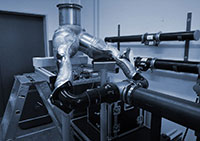• Air Filter and Air Box Testing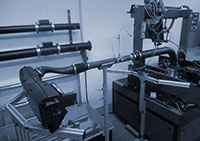• Intercooler Testing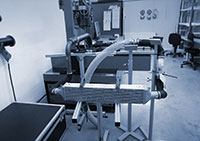# Terms & Definitions

### Stationary / Instationary Flow

• stationary flow: temporally constant velocity distribution (during observation period)
• Instationary flow: velocity distribution changes during the observation period (stochastic, periodic, transient)
• turbulent flow is instationary in principle but can approximately be seen as stationary if stochastic fractions of the motion compensate referring to time

### Compressible / Incompressible Flow

• incompressible: no changes of density between two observed spots of the flow
• the flow of a compressible medium (e.g. air) can be incompressible

### 1D Flow Straightener (one-dimensional)

A one-dimensional Flow Straightener (sometimes referred to as „Honeycomb”, because of the body structure) orients all velocity components of an arbitrary flow in one direction parallel to the cylinder axis. This means, all deviating momentum components are blocked by the flow straightener which causes reaction forces/torques in the bearing. Therefore the torque measured at the bearing represents the integral of the momenta in tangential direction over the Flow Straightener surface, referred to as Swirl. Besides the fact that there is no rotation (and therefore no undefined friction) of the device, the main advantage of this method of Swirl measurement is, that the quantity of interest is captured over the whole cross section at once and the result is valid without any assumptions concerning the flow (in contrast to the necessary “rigid disc”-assumption for winged wheels).

### 3D Flow Straightener (three-dimensional)

With the three-dimensional Flow Straightener the measurement principle described above is extended to all three dimensions of torque, delivering three components of swirl (Swirl around the cylinder axis and the two perpendicular components combined to Tumble) with one measurement. To achieve this, the cross section is transformed into a spheric shape and allows the flow to pass only in radial direction. The advantage of this method over all kinds of winged wheels (placed directly in the cylinder or – even worse – in a subsequent T-piece) are obvious in the measurement results, that show a good correlation e.g. with PIV results.

### Winged Wheels (paddle wheel, rotating ring, T-piece)

These kinds of swirl measurement devices are based on the idea to place a functionally shaped solid body pivot mounted in the flow, measure the rotational speed and draw a conclusion concerning the quantity of interest (swirl or tumble). This approach could only be successful if the rotation axis of such devices were congruent with the swirl axis, the swirl were homogenous (“rigid disc rotation”), the device could cover the whole cross section and the retardation by friction could be excluded or quantified – none of which is realistic. The additional assumption that flow components are conserved over long distances and even through changes of direction imposed by the piping – which would be essential for correct measurements using a T-piece – is at least rather optimistic. In modern equipment such devices should only be used to assure compatibility with existing databases.

### Particle Image Velocimetry

In PIV-measurements (particle image velocimetry) microscopic particles are illuminated by laser light sheet during two closely timed pulses and simultaneously recorded photographically. Using two cameras the spatial components of particle motion are obtained, from which the three-dimensional velocity field in the measuring plane is determined. The velocity fields can be visualized and are well suitable for the validation of computational fluid dynamics results.

# Formulas

### Mass Flow / Volume Flow

Quantity of a fluid that passes a cross section during a given time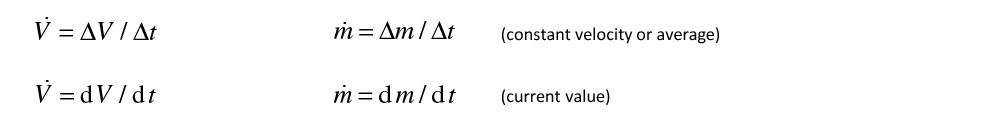with du (length increment at cross section A during dt):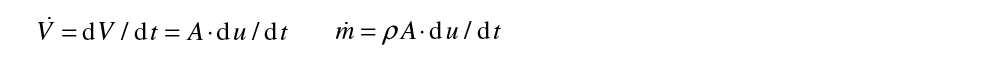→ for constant velocity c: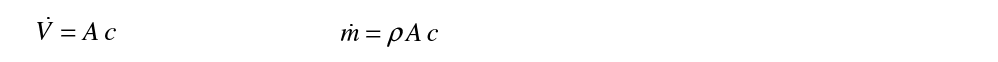### Energy Equation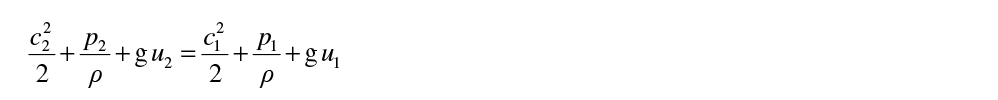### Energy Equation in Terms of Pressure

If differences in altitude can be neglected, the energy balance can be written as: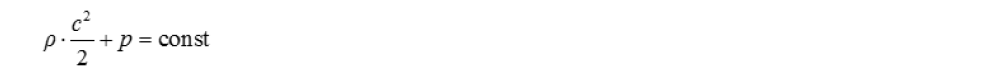using the terms

pst = p (static pressure)

pdvn = r c2 / 2 (dynamic pressure)

ptot (total pressure)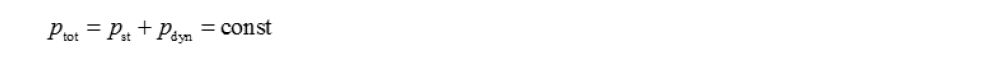in resting fluid (c = 0)### Leak Flow out of a Tank

(index 1: tank conditions; index 2: ambient conditions)
the second square root in the expression obtained for the mass flow is the function y* describing the leak flow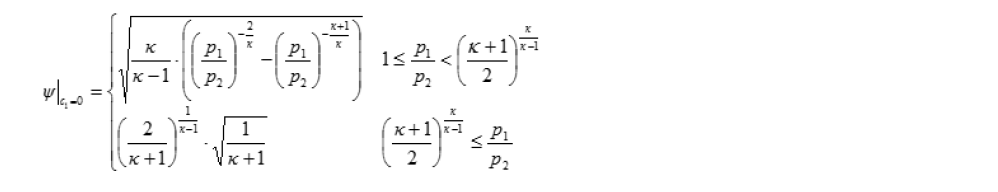### Effective Cross Section

Aeff is a characteristic value with the physical unit of an area and serves for the comparison of flows through different air ducts (ports) at a given pressure drop

Incompressible Flow (assumption)Compressible Flow### Tippelmann Swirl and Tumble Coefficient

Tippelmann Swirl and Tumble Coefficient are derived from the torque measured on the flow straighteners.

Tippelmann Swirl CoefficientTippelmann Tumble Coefficient and AnglePaddle Wheel measurement was the first attempt to determine a swirl proportional quantity to compare different ports. It is a

• comprehensible approach
• simple design

but

• the results are influenced by friction and
• no calibration is possible

Besides, this Paddle Wheel measurement obtains valid results only if:

• swirl axis and paddle wheel axis are congruent and
• the angular velocity in the paddle wheel rotation plane is distributed similar to a “rigid disc”.
• incompressible flow

Advantages of Momentum Principle (Torque based using Flow Straighteners)

• measuring in the section of interest (applies especially for tumble measurements)
• simultaneous measuring of moment of momentum of all 3 axes (x-; y-; z-axis)
• no friction and no wear
• easy calibration facilitates reliable results
• good correlation to PIV / LDA
• good correlation to CFD

# Momentum vs. PIV

Tippelmann Swirl and Tumble Coefficients derived from velocity fields generally show satisfying similarity to the respective values determined from torque measurements on flow straighteners. This illustrates the reliability of the Tippelmann method and shows that the two basic approaches can complement each other depending on the field of application.